# C programming session 01

30 de Aug de 2011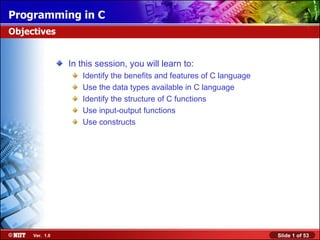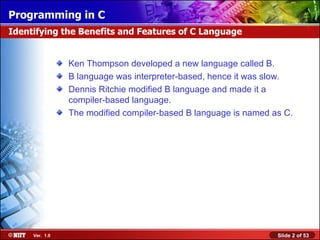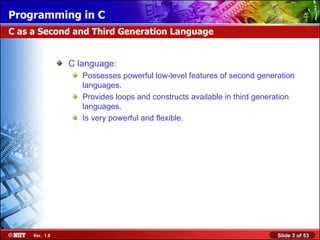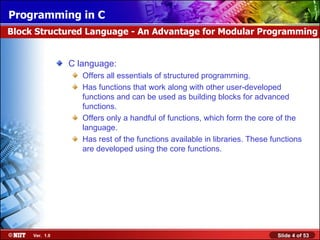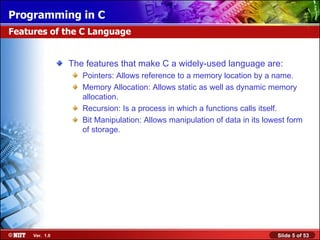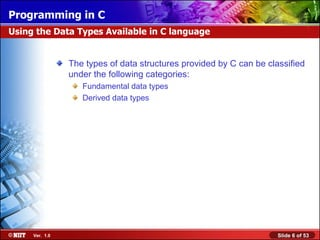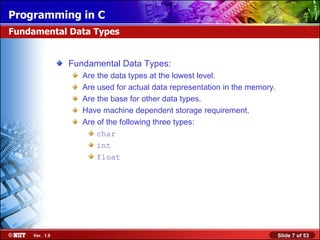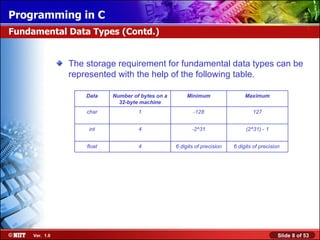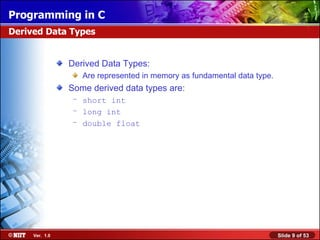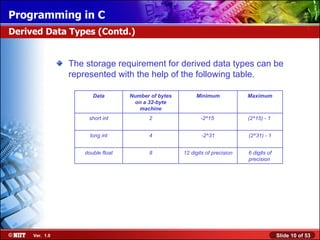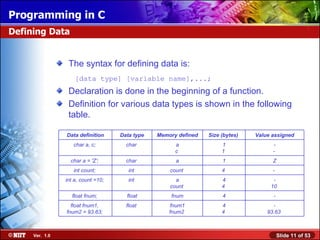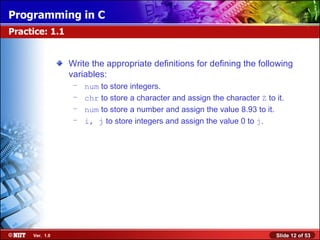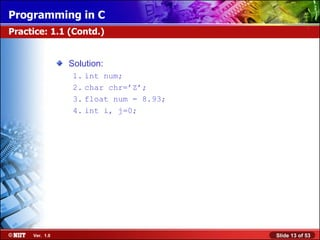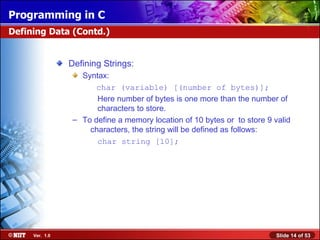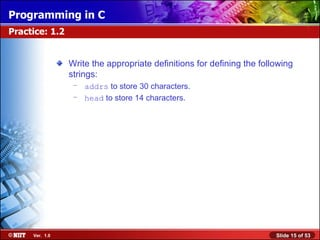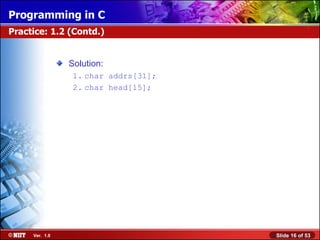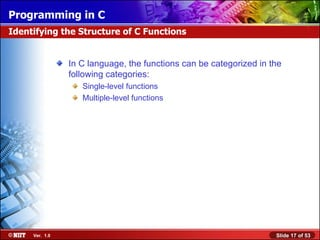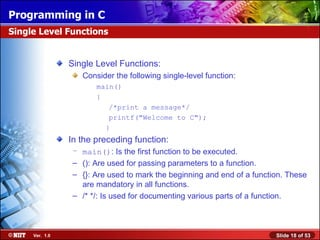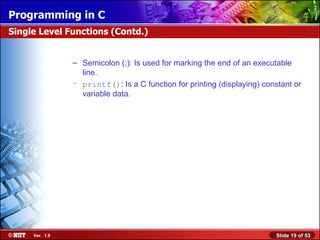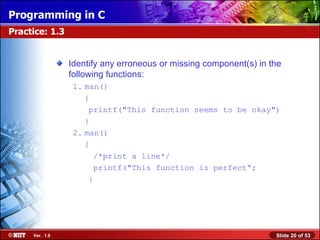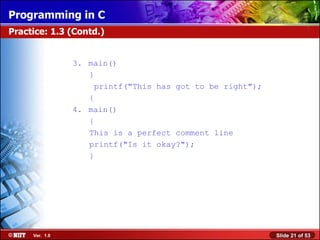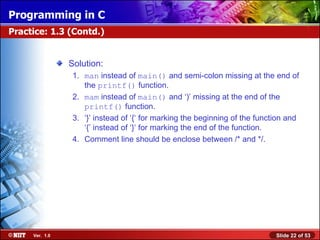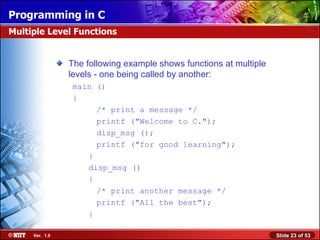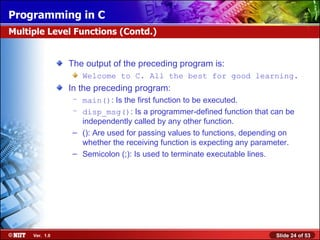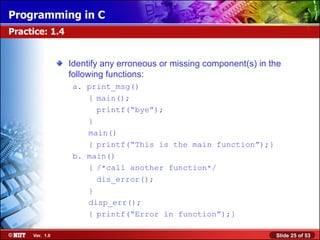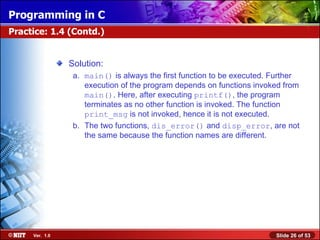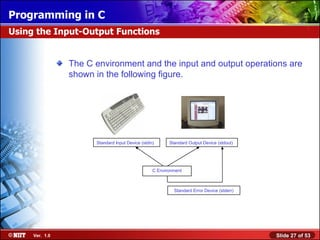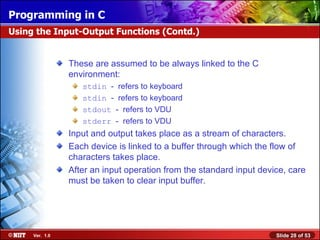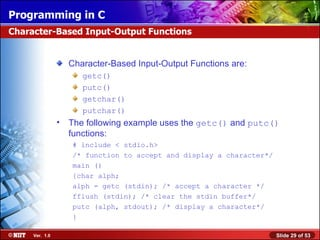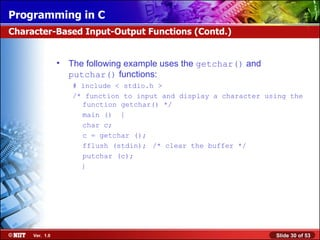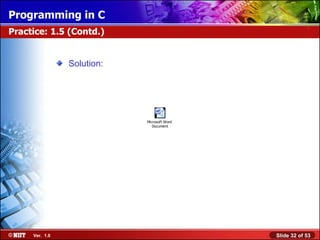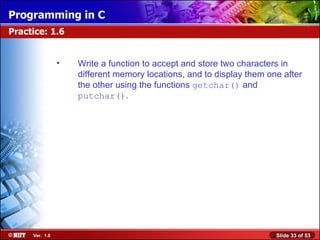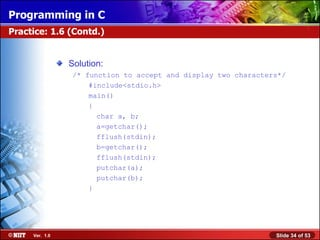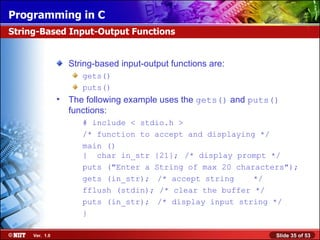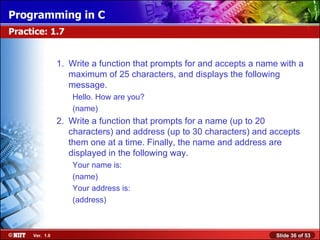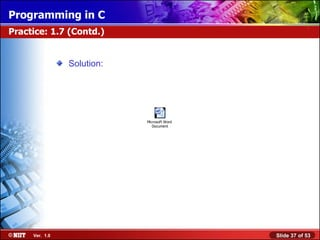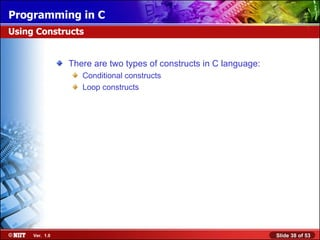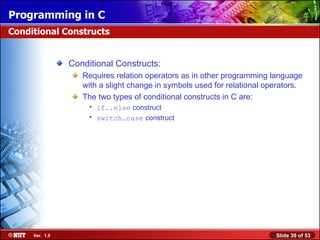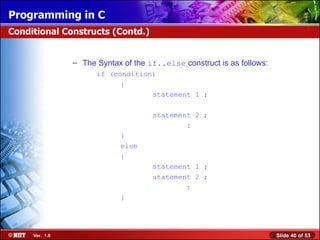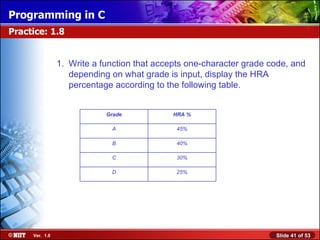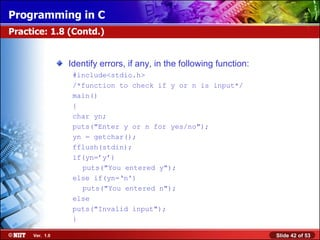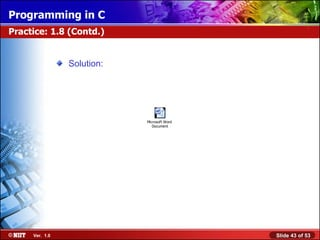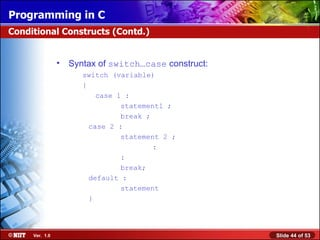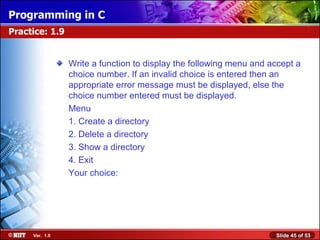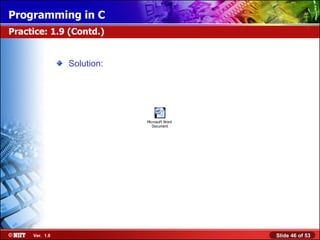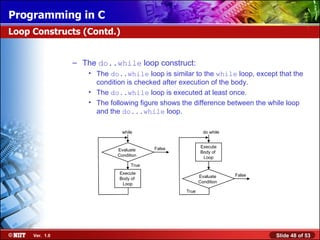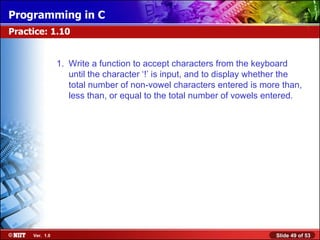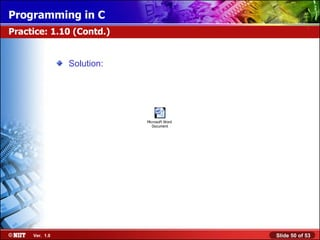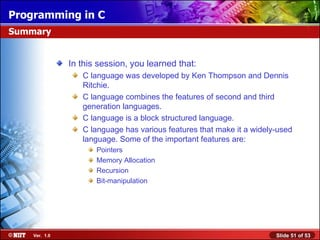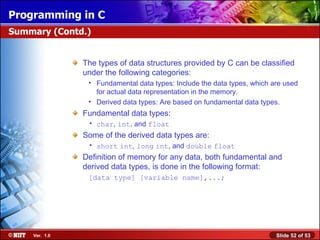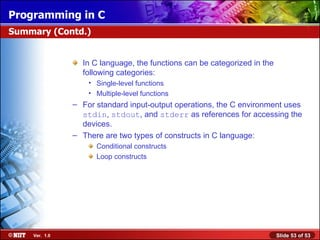1 de 53

• 1.
• 2.
• 3.
• 4.
• 5.
• 6.
• 7.
• 8.
• 9.
• 10.
• 11.
• 12.
• 13.
• 14.
• 15.
• 16.
• 17.
• 18.
• 19.
• 20.
• 21.
• 22.
• 23.
• 24.
• 25.
• 26.
• 27.
• 28.
• 29.
• 30.
• 31.
• 32.
• 33.
• 34.
• 35.
• 36.
• 37.
• 38.
• 39.
• 40.
• 41.
• 42.
• 43.
• 44.
• 45.
• 46.
• 47.
• 48.
• 49.
• 50.
• 51.
• 52.
• 53.

### Notas do Editor

1. Begin the session by explaining the objectives of the session.
2. Discuss the history of the C language.
3. The storage requirements for different data types are machine-dependent. There is a subtle difference between declaring and defining variables. The statement: char a; Is used to declare a variable a. Here, only the attributes of the variable are specified, not the contents. In contrast, the statement: char a = ‘A’ ; is used to define the contents of the variable a.
4. Derived data types modify the data according to the specific requirements. For example, if you need to store a integer value above 32,767 or below -32,767, you can use long int instead of int . the derived data type long increases the range of the int data type.
5. Use Slide 12 to test the student’s understanding on defining the variables.
6. Use Slide 15 to test the student’s understanding on defining the variables.
7. A C function is typically made up of blocks. A block is a set of C statements enclosed within braces. Variables are generally declared at the beginning of a function, but may also be declared at the beginning of a block. A C program is modular and structured. This allows for reusability of code.
8. Use Slide 20 to test the student’s understanding on functions.
9. Multiple-level functions are those functions where a function calls another function. Like in the slide, the main() function calls another function disp_msg() .
10. Use Slide 25 to test the student’s understanding on functions.
11. The getc() and getchar() functions are used to take a character from a stream. The getc() functions takes a stream as a parameter while the getchar() function does not take any parameter. Similarly, the putc() and putchar() function outputs a character to a stream. The putc() functions takes two parameters, the character to be output and the stream, while the putchar() function takes only one parameter i.e. the character to be output.
12. Use Slide 31 to test the student’s understanding on character-based input-output functions.
13. Use Slide 33 to test the student’s understanding on the getchar() and putchar() functions.
14. The gets() function is used to get a string from stdin . It collects a string terminated by a new line character from the input stream. It takes a constant string as a parameter. The puts() function copies the null-terminated string, which is passed as a parameter, to the standard output stream.
15. Use Slide 36 to test the student’s understanding on the gets() and puts() functions.
16. Explain the need for conditional constructs and loop constructs by taking appropriate examples.
17. Use Slide 41 to test the student’s understanding on the conditional constructs.
18. Explain the limitations of the switch…case construct. Tell the students that the switch…case construct cannot work with float variable. Also, you cannot specify an expression in the switch…case construct.
19. Use Slide 45 to test the student’s understanding on the conditional constructs.
20. The condition associated with the while loop is checked before entering the loop. Hence, there is a possibility that the loop is not executed at all. In the other case, the condition is checked after executing the loop once and for every interaction thereafter.
21. Use Slide 49 to test the student’s understanding on the loop constructs.
22. Use Slides 51, 52, and 53 to summarize the session.